Nuclear Physics MCQ Level – 2

# Nuclear Physics MCQ Level – 2

Test Description

## 10 Questions MCQ Test Topic wise Tests for IIT JAM Physics | Nuclear Physics MCQ Level – 2

Nuclear Physics MCQ Level – 2 for IIT JAM 2023 is part of Topic wise Tests for IIT JAM Physics preparation. The Nuclear Physics MCQ Level – 2 questions and answers have been prepared according to the IIT JAM exam syllabus.The Nuclear Physics MCQ Level – 2 MCQs are made for IIT JAM 2023 Exam. Find important definitions, questions, notes, meanings, examples, exercises, MCQs and online tests for Nuclear Physics MCQ Level – 2 below.
Solutions of Nuclear Physics MCQ Level – 2 questions in English are available as part of our Topic wise Tests for IIT JAM Physics for IIT JAM & Nuclear Physics MCQ Level – 2 solutions in Hindi for Topic wise Tests for IIT JAM Physics course. Download more important topics, notes, lectures and mock test series for IIT JAM Exam by signing up for free. Attempt Nuclear Physics MCQ Level – 2 | 10 questions in 45 minutes | Mock test for IIT JAM preparation | Free important questions MCQ to study Topic wise Tests for IIT JAM Physics for IIT JAM Exam | Download free PDF with solutions
 1 Crore+ students have signed up on EduRev. Have you?
Nuclear Physics MCQ Level – 2 - Question 1

### Suppose that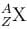decays by natural radioactivity in two stages to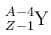the two stages would most likely be

Detailed Solution for Nuclear Physics MCQ Level – 2 - Question 1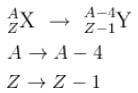Mass number reduces by 4 and number of protons by 1
option 1 says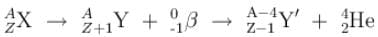∴  The first stage would be a β- decay and the second would be α emission.

The correct answer is:  β- emission with an anti neutrino  → α  emission

Nuclear Physics MCQ Level – 2 - Question 2

Detailed Solution for Nuclear Physics MCQ Level – 2 - Question 2

The correct answer is: random process

Nuclear Physics MCQ Level – 2 - Question 3

### Why do heavier nuclei have a greater ratio of neutrons to protons than lighter nuclei?

Detailed Solution for Nuclear Physics MCQ Level – 2 - Question 3

The correct answer is: to provide more attractive strong nuclear force to balance the repulsive electromagnetic force.

Nuclear Physics MCQ Level – 2 - Question 4

Which of the following nuclei has the largest Binding Energy/nucleon?

(Consider the most abundant isotope of each)

Detailed Solution for Nuclear Physics MCQ Level – 2 - Question 4

Nuclear Physics MCQ Level – 2 - Question 5

The 238U nucleus has a binding energy of about 7.6 MeV/nucleon. If the nucleus were to fission into 2 equal fragments, each would have a K.E. of just over 100 MeV. From this, it can be concluded that

Detailed Solution for Nuclear Physics MCQ Level – 2 - Question 5

The binding energy is the energy that keeps a nucleus together and makes its mass slightly different than its constituent particles.

If its masses were exactly the same as its constituent particles, its B.E would be 0 and it would be unstable.

Now, since the nucleus fissions into 2 equal fragmens.

∴  Each would have a mass number half of that of parent nucleus.

i.e.  A = 119 for each daughter nucleus. The difference in the Binding energies of the product and parent nucleus would be the K.E of the products.

If we assume that the daughter nucleus (each with A = 119 or A ~ 120) has a B.E of x per nucleon.

∴    –7.6 × 238 + x × 119 + x × 119 = 200 MeV
(–7.6 + x) 238 = 200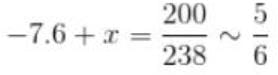–7.6 + x = 0.83
x = 7.6 + 0.83
x = 8.43
~ 8.5 MeV/nucleus

The correct answer is: Nuclei near A = 120 must be bound by about 8.5 MeV/nucleon.

Nuclear Physics MCQ Level – 2 - Question 6

When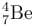transforms into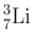does so by

Detailed Solution for Nuclear Physics MCQ Level – 2 - Question 6

Use the process of elimination.

An  α  decay would change the atomic number from 7. So it cannot be option 1. A beta decay requires 2 particles to be emitted e / e+ and r / r option 2 and 3 don’t satisfy this. This leaves us with the 4th option.

The correct answer is: Electron capture by the nucleus with emission of a neutrino.

Nuclear Physics MCQ Level – 2 - Question 7

Consider a heavy nucleus with spin 1/2 the magnitude of the ratio of the intrinsic magnetic moment of this nucleus to that of an electron is

Detailed Solution for Nuclear Physics MCQ Level – 2 - Question 7

Since the Nucleus and e  have the same spin.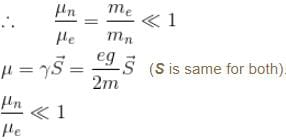Because mass of nucleus >> mass of e

The correct answer is: less than 1, because the nucleus has a mass much larger than that of the electron

Nuclear Physics MCQ Level – 2 - Question 8

A Uranium nucleus decays at rest into a Thorium nucleus, as shown.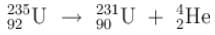Which of the following is true?

Detailed Solution for Nuclear Physics MCQ Level – 2 - Question 8

Since the U nucleus decays at rest.

∴  The products have equal and opposite value of momenta.

The product with greater mass will have lower K.E.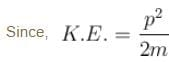The correct answer is: The Helium nucleus has more kinetic energy than the Thorium nucleus.

Nuclear Physics MCQ Level – 2 - Question 9

The primary source of sun’s energy is a series of thermo nuclear reactions in which the energy produced is c2  times the mass difference between

Detailed Solution for Nuclear Physics MCQ Level – 2 - Question 9

The reactions leading to production of energy in the sun are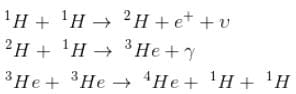i.e. 4 Hydrogen atoms and 1 Helium atom.
The correct answer is: 4 Hydrogen atoms and 1 Helium atom.

Nuclear Physics MCQ Level – 2 - Question 10

Internal conversion is a process where by an excited nucleus transfers its energy directly to one of the most tightly bound atomic electrons, causing the electron to be ejected from the atom and leaving the atom in an excited state. The most probable process after an internal conversion electron is ejected from an atom with a high atomic number is that the

Detailed Solution for Nuclear Physics MCQ Level – 2 - Question 10

Since the internal conversion leaves the atom in the excited state (and not the nucleus).

∴  option 2 seems most probable

The correct answer is: atom emits one or several X-rays

## Topic wise Tests for IIT JAM Physics

217 tests
 Use Code STAYHOME200 and get INR 200 additional OFF Use Coupon Code
Information about Nuclear Physics MCQ Level – 2 Page
In this test you can find the Exam questions for Nuclear Physics MCQ Level – 2 solved & explained in the simplest way possible. Besides giving Questions and answers for Nuclear Physics MCQ Level – 2, EduRev gives you an ample number of Online tests for practice

217 tests